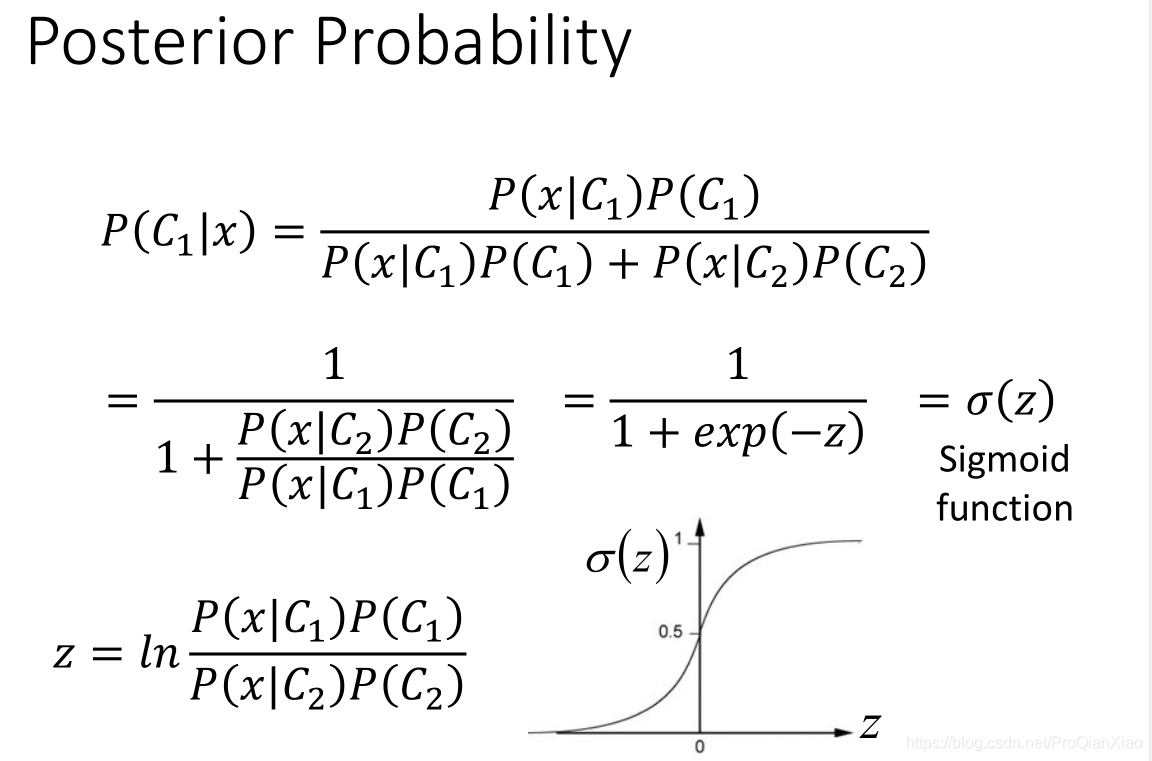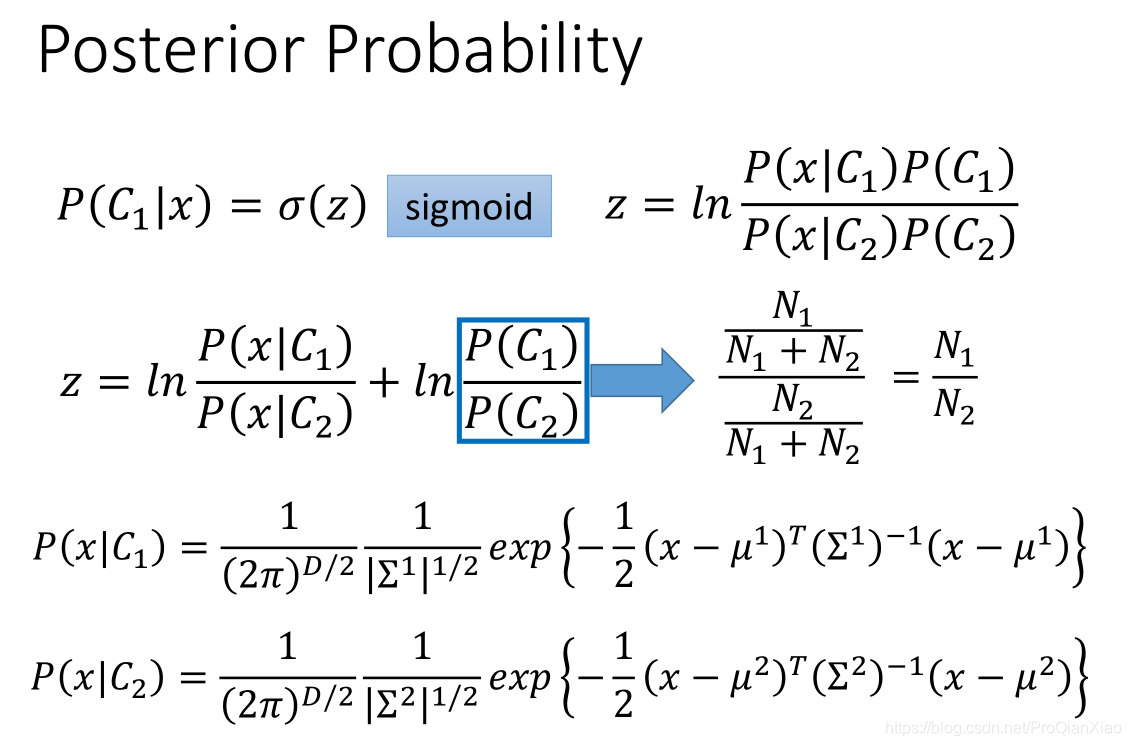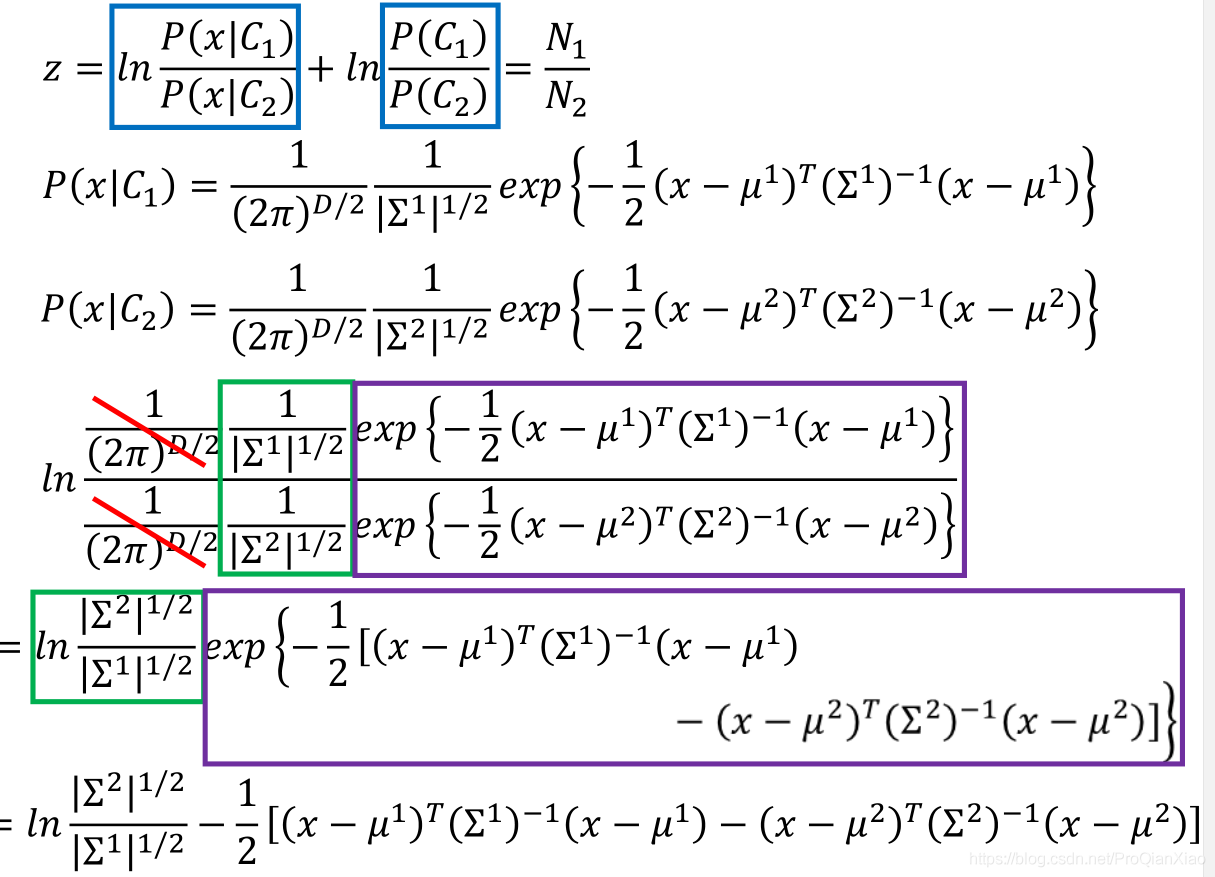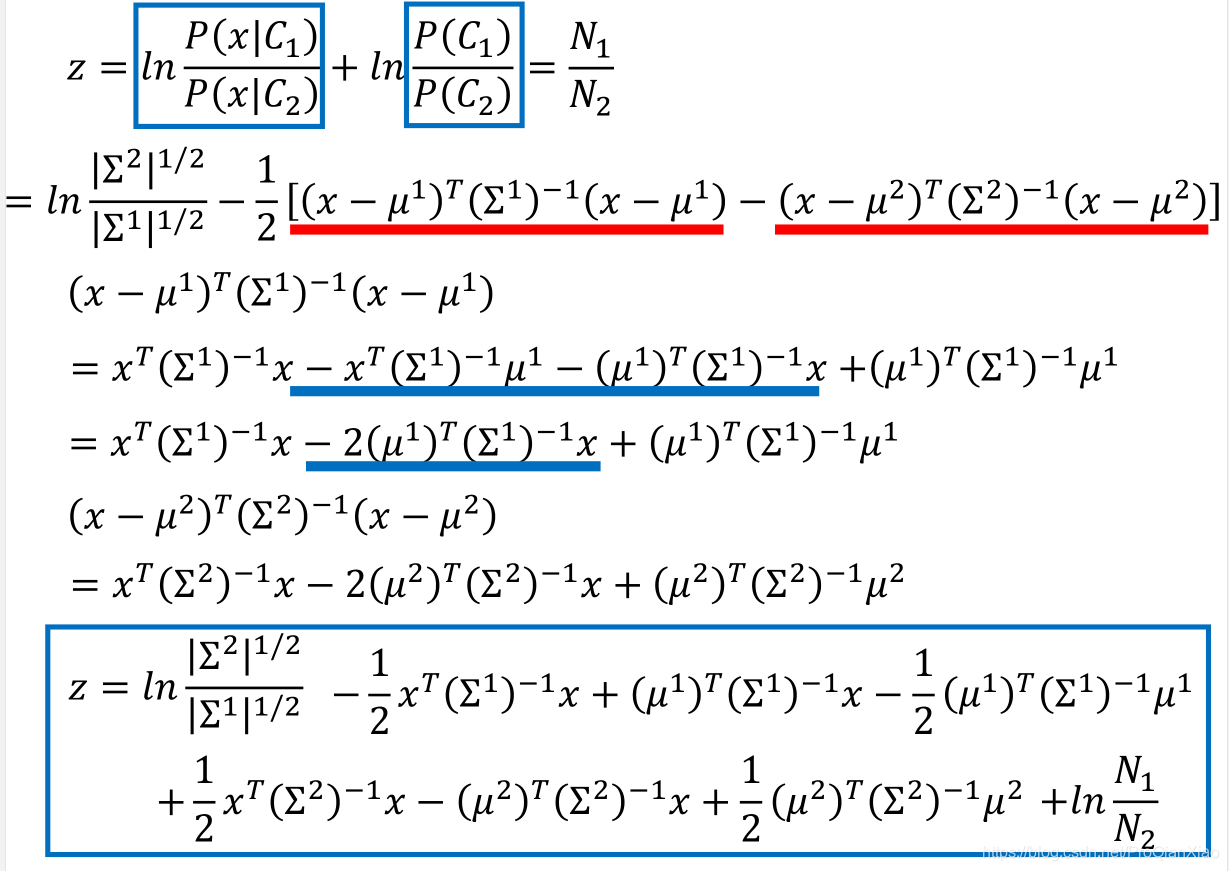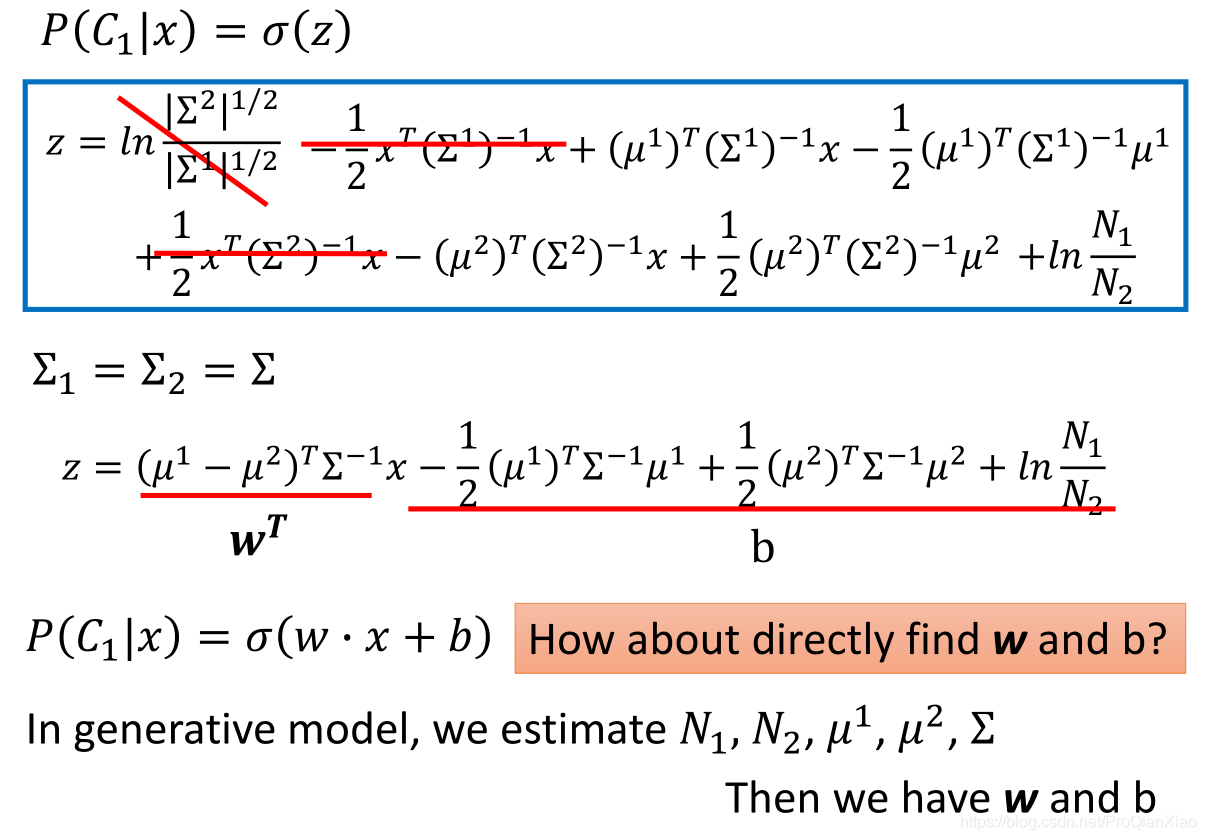## 理论基础

$P(A|B)=\frac{P(B|A)P(A)}{P(B)} \tag{1}$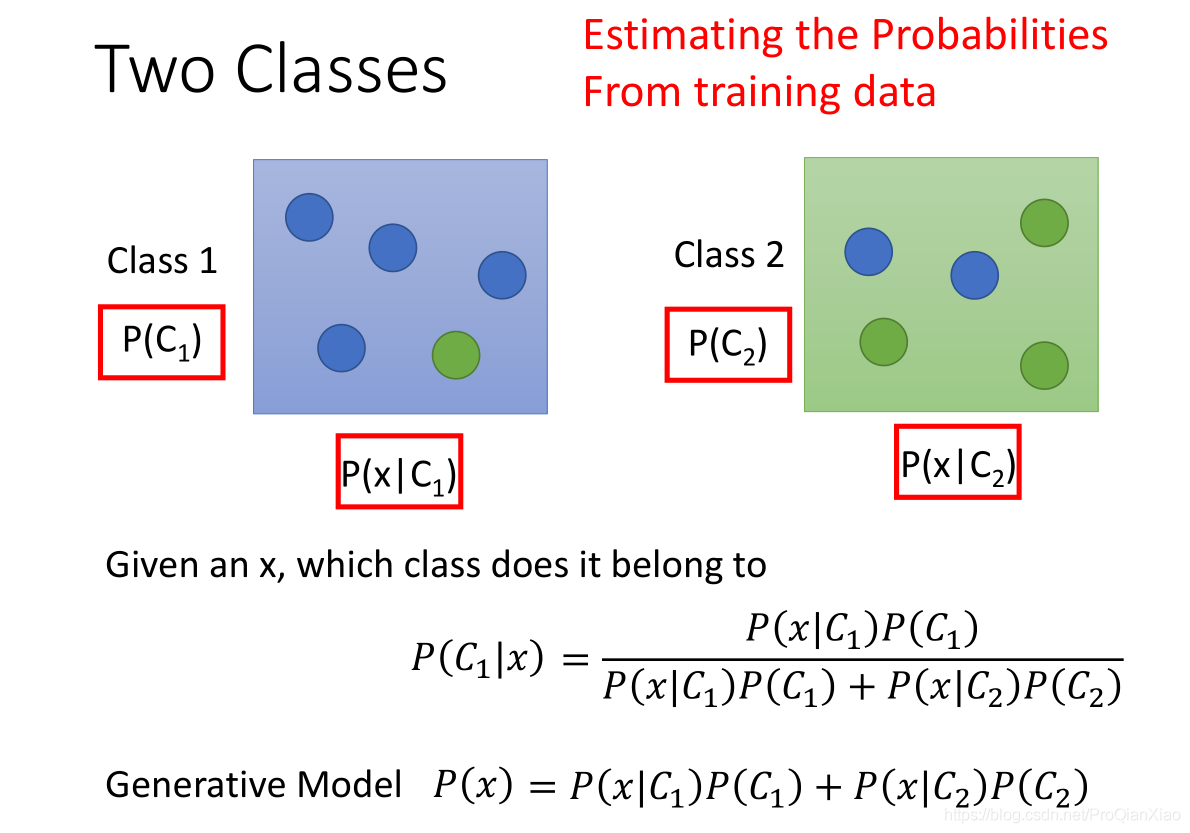$P(x) = P(x|C1)P(C1) + P(x|C2)P(C2)$

$P=(x_1,x_2,...,x_n)=f_D(x_1,x_2,...,x_n|\theta)$

$lik(\theta)=f_D(x_1,x_2,...,x_n|\theta)$

## 实例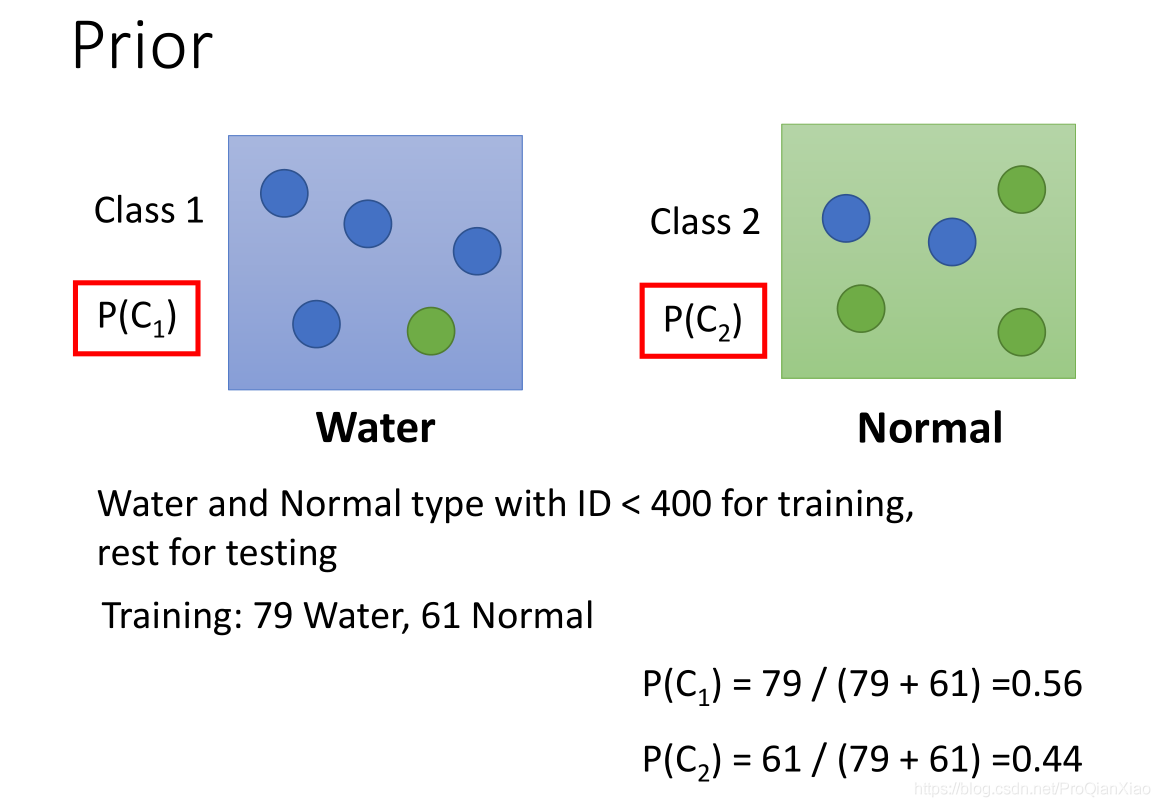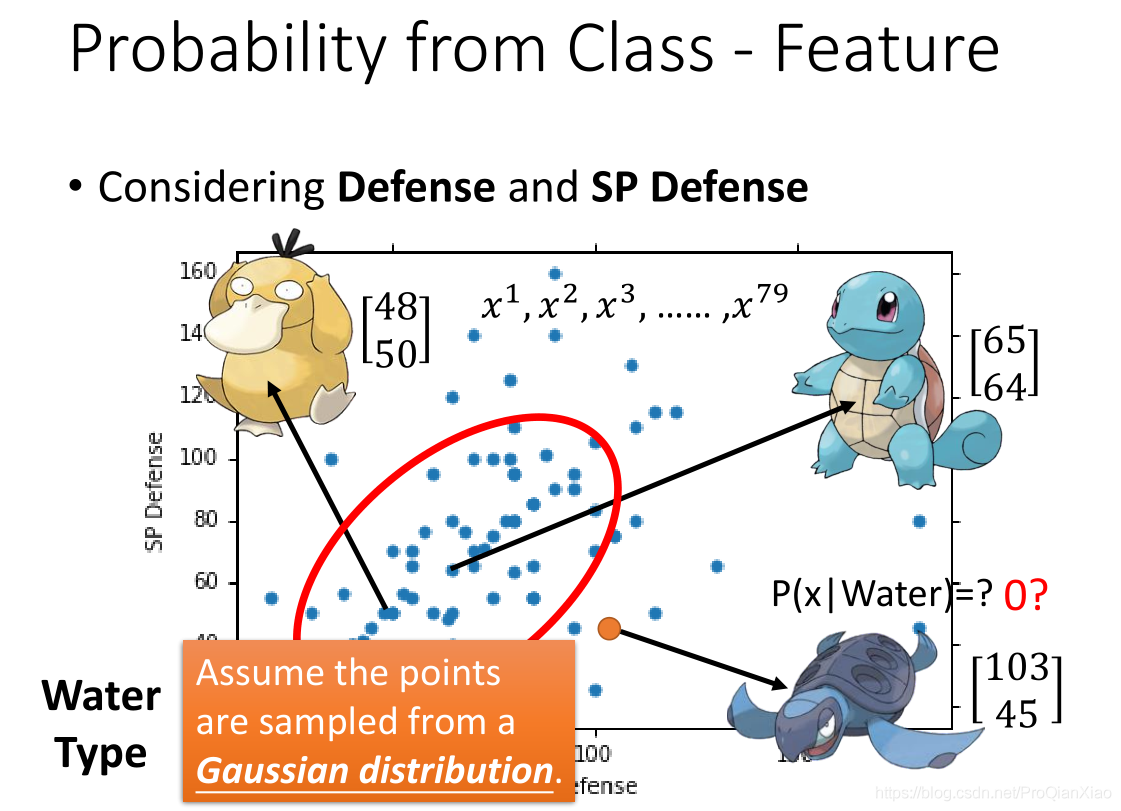• 均值表征的是各维变量的中心
• 协方差矩阵的对角线上的两个元素，决定了整个高斯曲面在某一维度上的“跨度”，方差越大，“跨度”越大
• 协方差矩阵的斜对角线上面的两个元素，表征的是各维变量之间的相关性，其数值大于零说明x与y呈正相关，其值越大，正相关程度越大，小于零则是负相关，否则则是不相关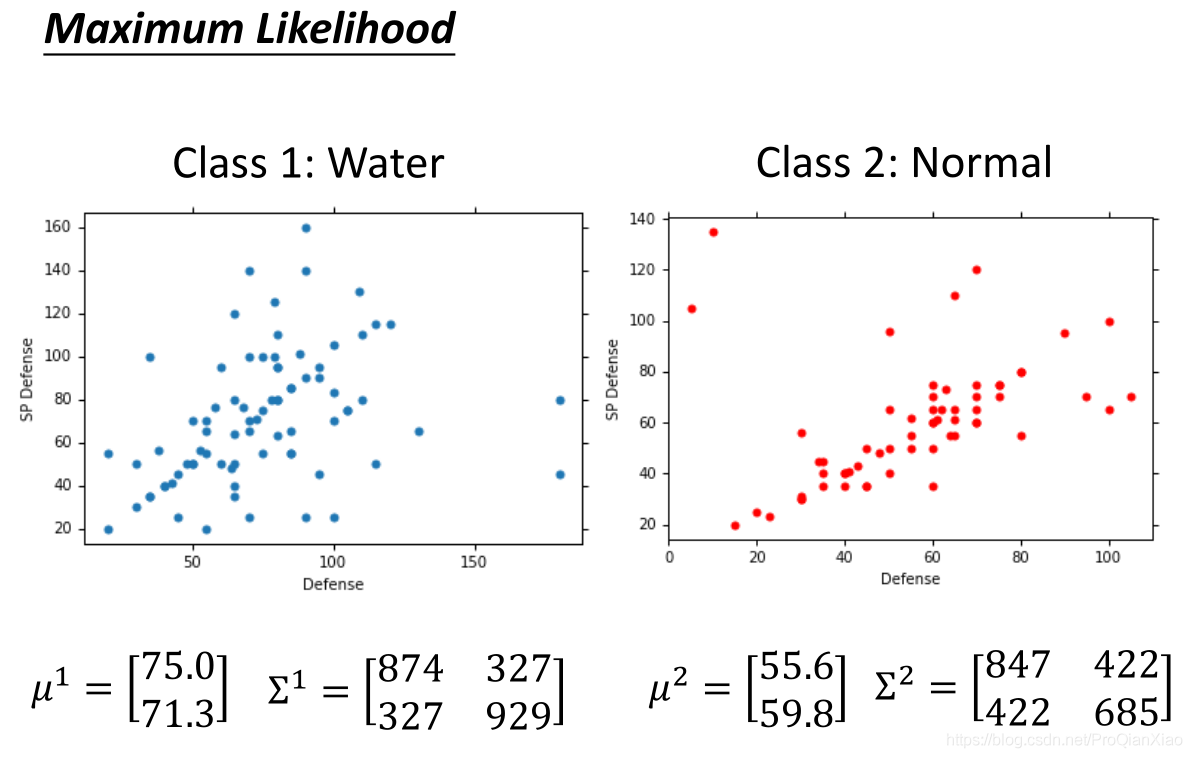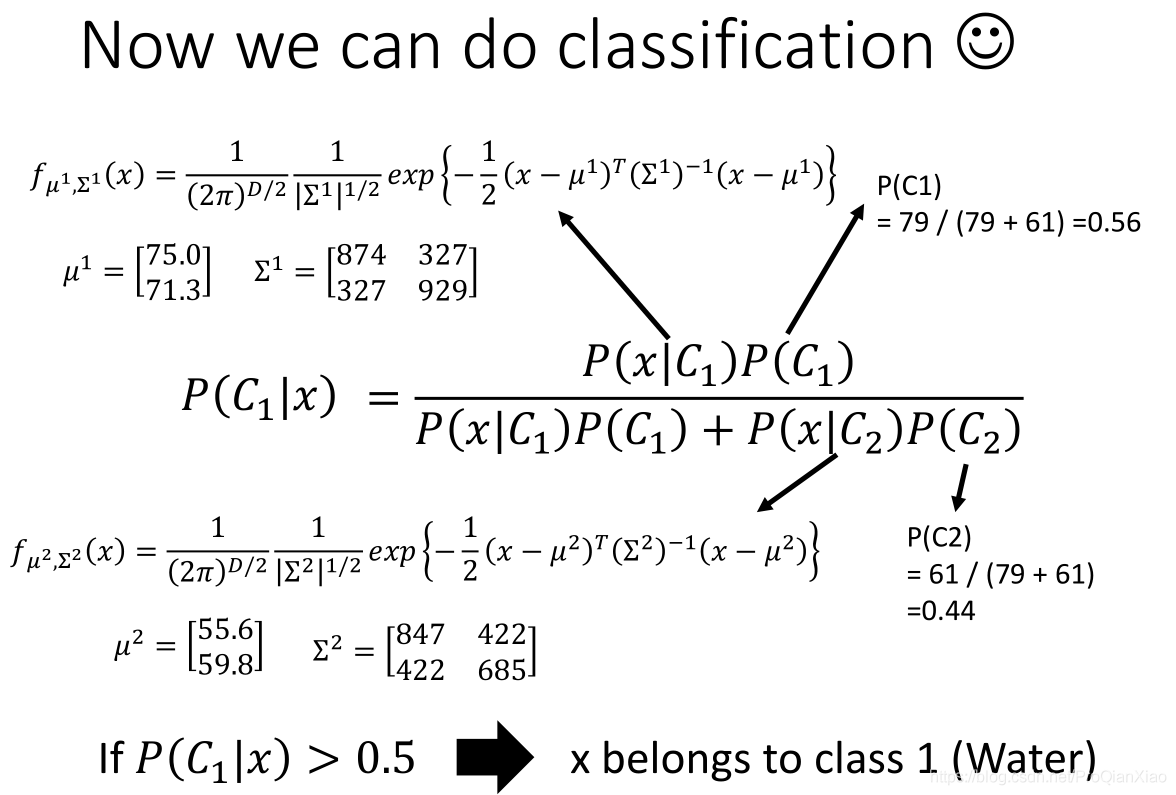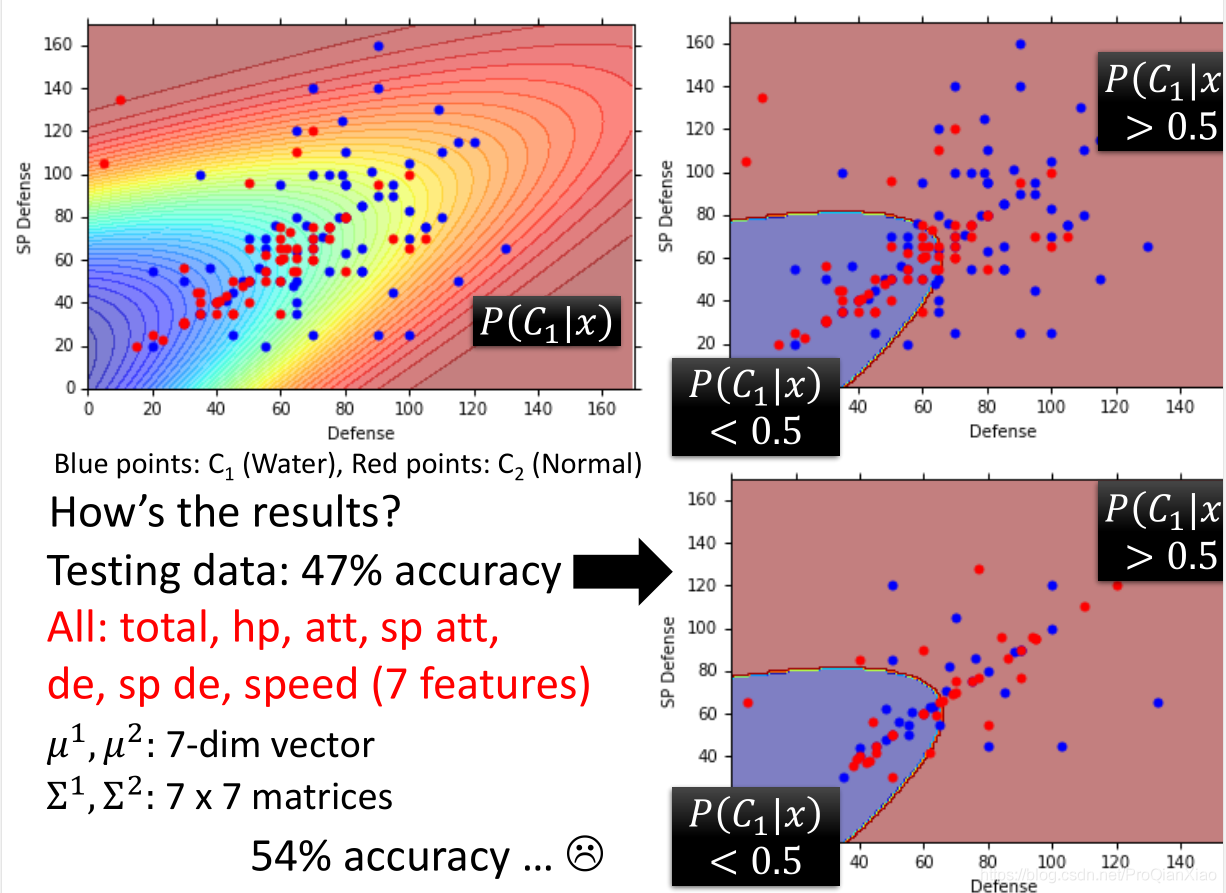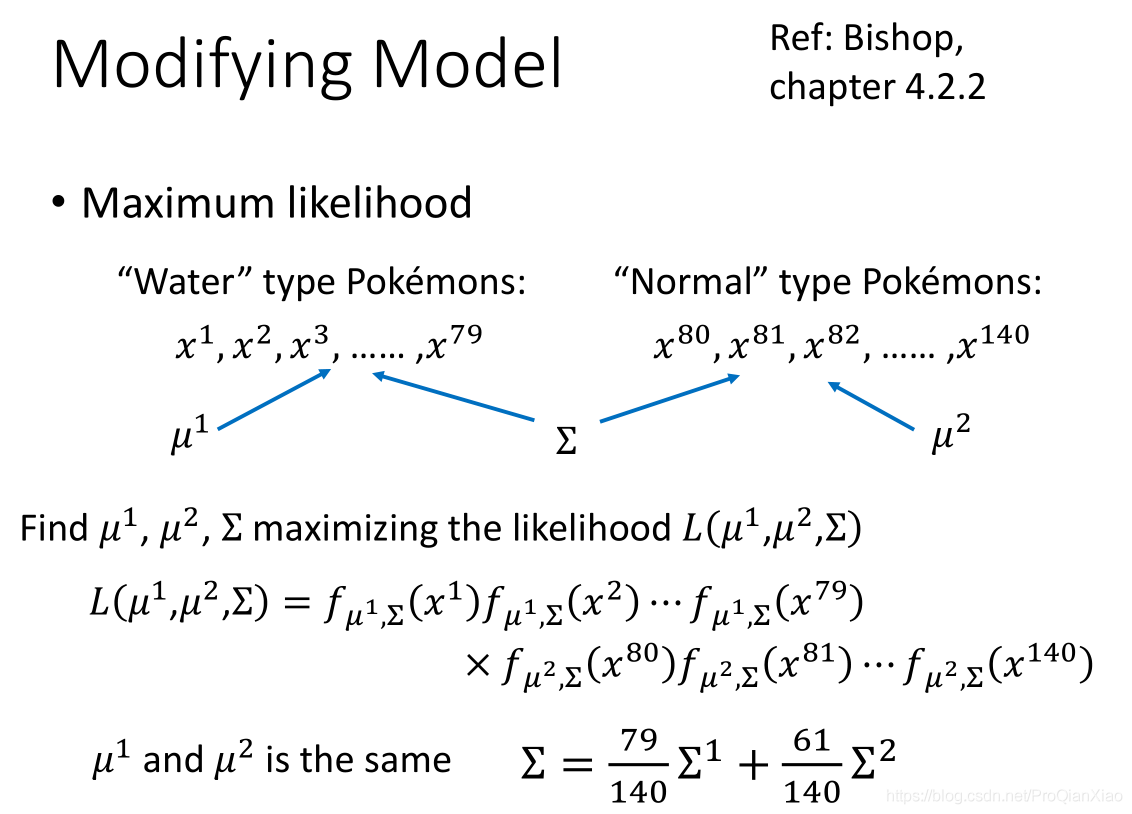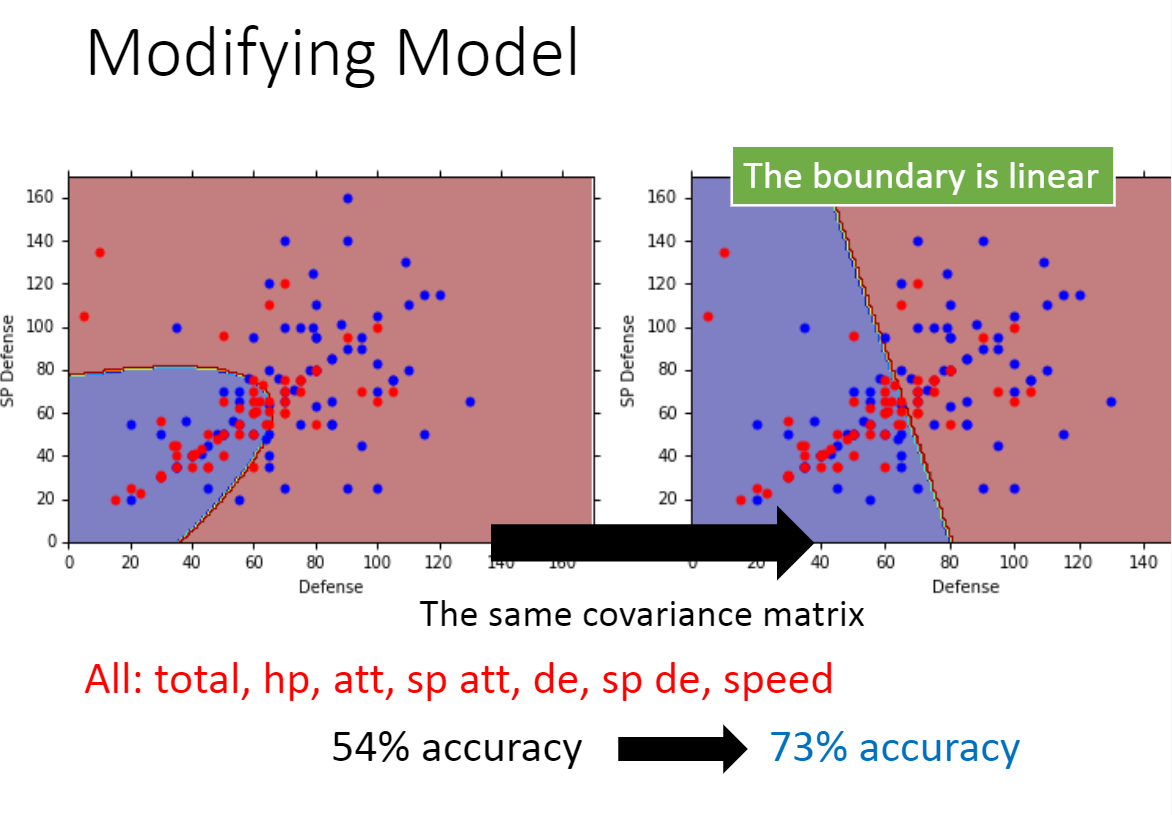## 总结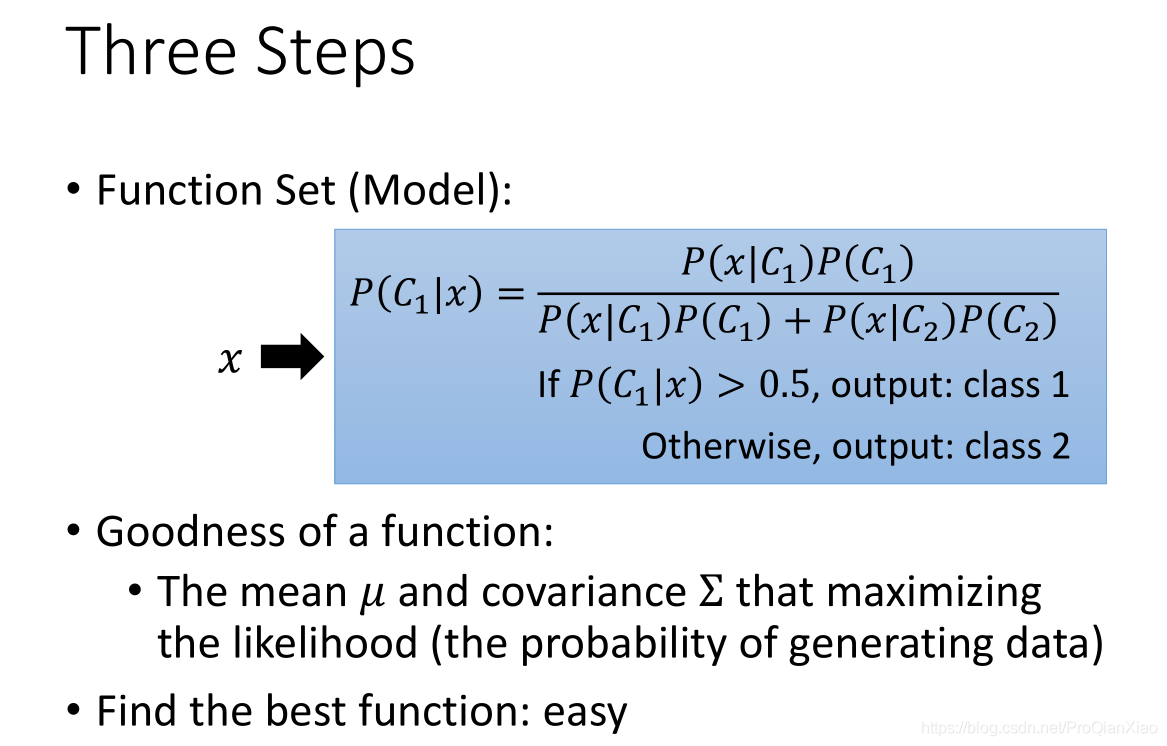## 后续